Example Questions

← Previous 1 3

Jack and Jill have saved up $3500 for a vacation to Bermuda. Package Deal A costs$2500 plus an additional 30% tax. Package Deal B costs $2400 with an additional 3/9 of the price added for airfare. Package deal C costs$3225 with an included 10% tax. Package deal D costs $1800 per person. Which package deal will save them the most money? Possible Answers: D B A C Correct answer: B Explanation: Calculate the price of each Package Deal. A =$2500(1.3) = $3250, B =$2400 (1+3/9) = $3200. C =$3225, D = $3600. Remember that 1 = 9/9 for fraction addition. Package deal C’s wording is tricky, the tax is already included. Package Deal B is the best deal. Example Question #1 : How To Find Percentage Tim Duncan made 14 out of 16 free throws. Tony Parker made 9 out of 10 free throws. Danny Green made 7 out of 8 free throws. Whose shooting percentage was the best? Possible Answers: Tim and Danny were both equally the best Tim Duncan Danny Green Tony Parker All three players were equally the best Correct answer: Tony Parker Explanation: For Tim, his percentage was .875 or 87.5%. For Tony, his percentage was .90 or 90%. For Danny, his percentage was also .875 or 87.5%. Thus Tony was the best. Example Question #3 : Other Percentage Johnny earned 5 % commission on a car he sold for$25,000.  How much money did he make?

None of the answers are correct

$2,000$1,500

$1,250$2,500

$1,250 Explanation:$ sales x % Commission = $Commission 25000 x 0.05 = 1250 Example Question #4 : Other Percentage Joey buys a sweatshirt for$35 that was originally sold for $50. What is the percent discount? Possible Answers: 15 % 30 % 50 % 70 % 20 % Correct answer: 30 % Explanation: Amount of discount = original price – new price % discount = amount of discount ÷ original price % discount = 15/50 = 0.3 or 30% Example Question #5 : Other Percentage Bryan has white socks and blue socks in his drawer, in a ratio of 4 : 7. What percent of his socks are white? Possible Answers: 30% 57% 75% 64% 36% Correct answer: 36% Explanation: You divide the number of blue socks, 4, by the number of total socks, 11, giving you 36%. Example Question #6 : Other Percentage There are 9 marbles in a box, and 4 of them are red. What percentage of the marbles in the box are NOT red? Possible Answers: 45% 55% 44% 99% 50% Correct answer: 55% Explanation: There are 5 non-red marbles and 9 total marbles. 5/9 = 0.555, which is about 55% Example Question #151 : Arithmetic What number is 130% of 50? Possible Answers: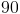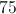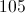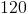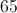Correct answer:Explanation: Verbal cues are "is" and "of." Is means equals and of means multiplication. So the equation to solve becomes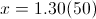which equals 65. Example Question #1 : Other Percentage Sally earns 3% commission on a house she sold for$150,000.  How much money did she earn?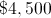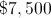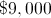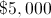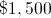Explanation: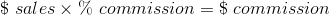So the equation to solve becomes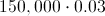or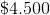earned.

Example Question #2 : How To Find Percentage

What number is 125% of 160?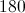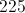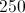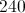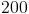Explanation:

Verbal cues include "is" and "of."  "Is" means equals and "of" means multiply.

So the equation to solve becomes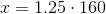or.

Example Question #10 : Other Percentage

A high school student spends a total of 90 minutes per day studying for the ACT, Monday through Friday, then 2 hours a day on the weekend.  What percent of their week is spent studying for the ACT?

5.5%

8%

6.25%

6.8%

7.125%

6.8%

Explanation:

The number of minutes in a week is: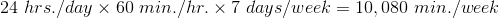The number of minutes spent studying is: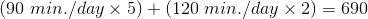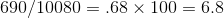6.8%

← Previous 1 3